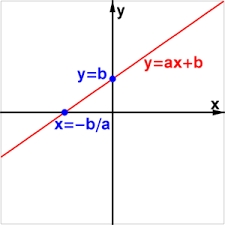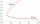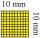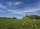# Suppose

Suppose you know that the length of a line segment is 15, x2=6, y2=14 and x1= -3. Find the possible value of y1. Is there more than one possible answer? Why or why not?

y11 =  26
y12 =  2

### Step-by-step explanation:Did you find an error or inaccuracy? Feel free to write us. Thank you!

Showing 1 comment:Matematik
we make circle k with centre S(x2,y2) and radius r = 15 . Then we make vertical line x= -3 . It make two intersections with circle k thus solutions are two: y11,y12.Tips to related online calculators
Line slope calculator is helpful for basic calculations in analytic geometry. The coordinates of two points in the plane calculate slope, normal and parametric line equation(s), slope, directional angle, direction vector, the length of the segment, intersections of the coordinate axes, etc.
Looking for help with calculating roots of a quadratic equation?
Do you have a linear equation or system of equations and looking for its solution? Or do you have a quadratic equation?
Do you want to convert length units?
Pythagorean theorem is the base for the right triangle calculator.

#### You need to know the following knowledge to solve this word math problem:

We encourage you to watch this tutorial video on this math problem:

## Related math problems and questions:

• ProveProve that k1 and k2 are the equations of two circles. Find the equation of the line that passes through the centers of these circles. k1: x2+y2+2x+4y+1=0 k2: x2+y2-8x+6y+9=0
• Intersections 3Find the intersections of the circles x2 + y2 + 6 x - 10 y + 9 = 0 and x2 + y2 + 18 x + 4 y + 21 = 0
• Trapezoid 15Area of trapezoid is 266. What value is x if bases b1 is 2x-3, b2 is 2x+1 and height h is x+4
• Curve and lineThe equation of a curve C is y=2x² -8x+9, and the equation of a line L is x+ y=3 (1) Find the x coordinates of the points of intersection of L and C. (2) Show that one of these points is also the stationary point of C?
• Sphere equationObtain the equation of sphere its centre on the line 3x+2z=0=4x-5y and passes through the points (0,-2,-4) and (2,-1,1).
• Find the 15Find the tangent line of the ellipse 9 x2 + 16 y2 = 144 that has the slope k = -1Which of the points belong function f:y= 2x2- 3x + 1 : A(-2, 15) B (3,10) C (1,4)
• Non linear eqsSolve the system of non-linear equations: 3x2-3x-y=-2 -6x2-x-y=-7
• Circle - AGFind the coordinates of circle and its diameter if its equation is: x2 + y2 - 6x-4y=36
• If x=4If x=4 and y=-3, what is the value of the expression x² + 2xy - 4x + 3y + y²?
• Sphere from tree pointsEquation of sphere with three point (a,0,0), (0, a,0), (0,0, a) and center lies on plane x+y+z=a
• CenterCalculate the coordinates of the circle center: x2 -4x + y2 +10y +25 = 0
• Square areaComplete the table and then draw each square. Provide exact lengths. Describe any problems you have. Side Length Area Square 1 1 unit2 Square 2 2 units2 Square 3 4 units2
• A Cartesian framework1. In a Cartesian framework, the functions f and g we know that: the function (f) is defined by f (x) = 2x ^ 2, the function (g) is defined by g (x) = x + 3, the point (O) is the origin of the reference, point (C) is the point of intersection of the graph
• Find the 13Find the equation of the circle inscribed in the rhombus ABCD where A[1, -2], B[8, -3] and C[9, 4].
• Find the 5Find the equation of the circle with center at (1,20), which touches the line 8x+5y-19=0
• Distance problem 2A=(x,2x) B=(2x,1) Distance AB=√2, find value of x